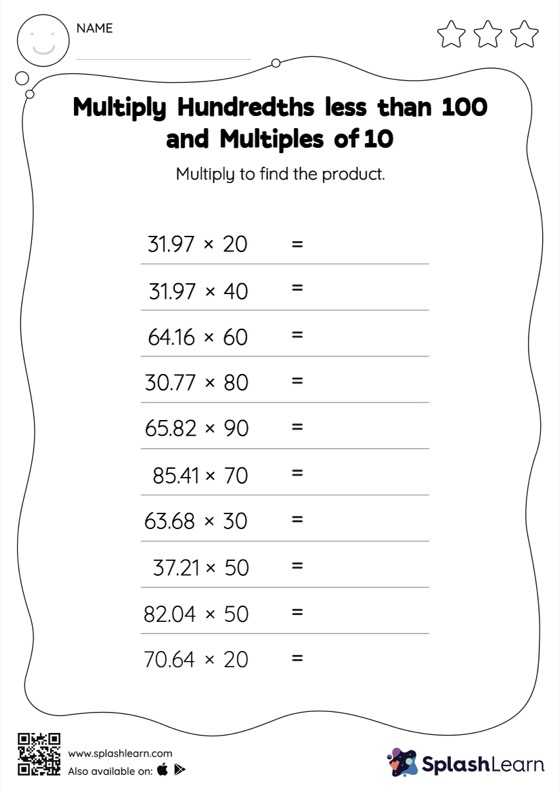# Multiply Hundredths less than 100 and Multiples of 10: Horizontal Multiplication Worksheet

Home > Multiply Hundredths less than 100 and Multiples of 10: Horizontal MultiplicationThis worksheet invites students to multiply hundredths less than 100 and multiples of 10, which helps them become proficient in multiplication. Students understand that multiplying a decimal by a number that ends in zeros causes the decimal point to be placed in the product as many places from the right as the number of decimal digits in the multiplicand. In multiply hundredths less than 100 and multiples of 10 worksheet, they practice this idea in great detail.